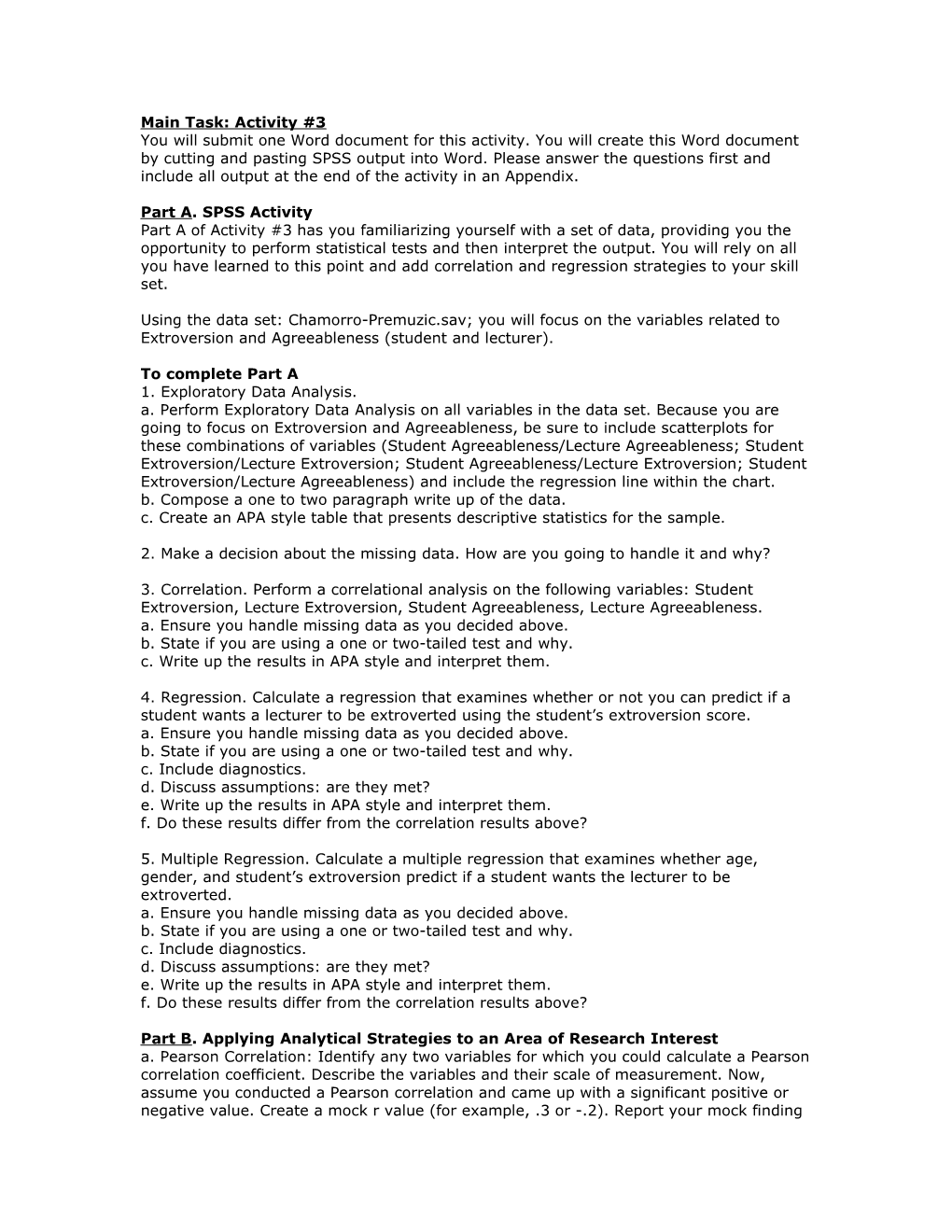You will submit one Word document for this activity. You will create this Word document by cutting and pasting SPSS output into Word. Please answer the questions first and include all output at the end of the activity in an Appendix.
Part A. SPSS Activity
Part A of Activity #3 has you familiarizing yourself with a set of data, providing you the opportunity to perform statistical tests and then interpret the output. You will rely on all you have learned to this point and add correlation and regression strategies to your skill set.
Using the data set: Chamorro-Premuzic.sav; you will focus on the variables related to Extroversion and Agreeableness (student and lecturer).
To complete Part A
1. Exploratory Data Analysis.
a. Perform Exploratory Data Analysis on all variables in the data set. Because you are going to focus on Extroversion and Agreeableness, be sure to include scatterplots for these combinations of variables (Student Agreeableness/Lecture Agreeableness; Student Extroversion/Lecture Extroversion; Student Agreeableness/Lecture Extroversion; Student Extroversion/Lecture Agreeableness) and include the regression line within the chart.
b. Compose a one to two paragraph write up of the data.
c. Create an APA style table that presents descriptive statistics for the sample.
2. Make a decision about the missing data. How are you going to handle it and why?
3. Correlation. Perform a correlational analysis on the following variables: Student Extroversion, Lecture Extroversion, Student Agreeableness, Lecture Agreeableness.
a. Ensure you handle missing data as you decided above.
b. State if you are using a one or two-tailed test and why.
c. Write up the results in APA style and interpret them.
4. Regression. Calculate a regression that examines whether or not you can predict if a student wants a lecturer to be extroverted using the student’s extroversion score.
a. Ensure you handle missing data as you decided above.
b. State if you are using a one or two-tailed test and why.
c. Include diagnostics.
d. Discuss assumptions: are they met?
e. Write up the results in APA style and interpret them.
f. Do these results differ from the correlation results above?
5. Multiple Regression. Calculate a multiple regression that examines whether age, gender, and student’s extroversion predict if a student wants the lecturer to be extroverted.
a. Ensure you handle missing data as you decided above.
b. State if you are using a one or two-tailed test and why.
c. Include diagnostics.
d. Discuss assumptions: are they met?
e. Write up the results in APA style and interpret them.
f. Do these results differ from the correlation results above?
Part B. Applying Analytical Strategies to an Area of Research Interest
a. Pearson Correlation: Identify any two variables for which you could calculate a Pearson correlation coefficient. Describe the variables and their scale of measurement. Now, assume you conducted a Pearson correlation and came up with a significant positive or negative value. Create a mock r value (for example, .3 or -.2). Report your mock finding in APA style (note the text does not use APA style) and interpret the statistic in terms of effect size and R2 while also taking into account the third variable problem as well as direction of causality.
b. Spearman’s Correlation: Identify any two variables for which you could calculate a Spearman’s correlation coefficient. Describe the variables and their scale of measurement. Now, assume you conducted a correlation and came up with a significant positive or negative value. Create a mock r value (for example, .3 or -.2). Report your mock finding in APA style and interpret the statistic in terms of effect size and R2 while also taking into account the third variable problem as well as direction of causality.
c. Partial Correlation vs. Semi-Partial Correlation: Identify any three variables for which you may be interested in calculating either a partial or semi-partial correlation coefficient. Compare/contrast these two types of analyses using your variables and research example. Which would you use and why?
d. Simple Regression: Identify any two variables for which you could calculate a simple regression. Describe the variables and their scale of measurement. Which variable would you include as the predictor variable and which as the outcome variable? Why? What would R2 tell you about the relationship between the two variables?
e. Multiple Regression: Identify at least 3 variables for which you could calculate a multiple regression. Describe the variables and their scale of measurement. Which variables would you include as the predictor variable and which as the outcome variable? Why? Which regression method would you use and why? What would R2 and adjusted R2 tell you about the relationship between the variables?
f. Logistic Regression: Identify at least 3 variables for which you could calculate a logistic regression. Describe the variables and their scale of measurement. Which variables would you include as the predictor variable and which as the outcome variable? Why? Which regression method would you use and why? What would the output tell you about the relationship between the variables?